# ISEE Lower Level Math : How to find the area of a square

## Example Questions

← Previous 1 3 4 5

### Example Question #72 : Squares

If the perimeter of a square is 18 cm, what must the area of this square be?

81cm2

18cm2

20.25cm2

16cm2

25cm2

20.25cm2

Explanation:

Remember that a square has four sides, each side with equal lengths. If a square has a perimeter of 18 cm, that means that each side has a length of

18 ÷ 4 =

4.5 cm.

The formula for the area of a square is length x width or length squared (length x length), since each side of a square has the same length.

So,

4.5 x 4.5 =

20.25cm2

### Example Question #1 : How To Find The Area Of A Square

What is the area of a square that has a side with a length of 9 cm?

81

63

36

18

81

Explanation:

The area of a square can be found using the same equation as the area of a rectangle - length times width.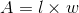However, in the case of a square, all of the sides are of equal length. Therefore, the area of a square can be found using the equation: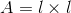or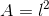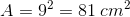### Example Question #3 : How To Find The Area Of A Square

What is the area of a square that has a side with a length of 10 in.?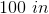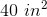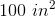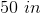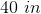Explanation:

To calculate the area of a square, you need to multiply the length by the width. By definition, a square has 4 equal sides. So, by knowing the length of one side of the square, you know the length measurement and the width measurement.

Since the length of one side of this square is 10 in., you would multiply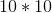to get the answer.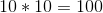Because area is the number of squares that would be used to cover the inside the shape, it is given in units2. As a result, the answer is 100 in.2.

### Example Question #4 : How To Find The Area Of A Square

The perimeter of a square is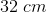.  What is the area?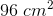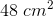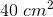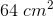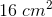Explanation:

The perimeter of a square is given by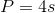.  Here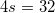, so divide by four to get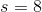.

The area of a square is given by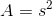. Substitute the side length obtained from the perimeter equation to get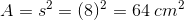.

### Example Question #5 : How To Find The Area Of A Square

The perimeter of a square is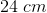.  What is the area?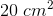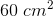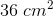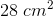Explanation:

The perimeter of a square is given by,  or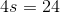. Divide by four to get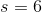.

The area of a square is given by. Substitute the side length obtained from the perimeter equation to get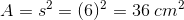.

### Example Question #6 : How To Find The Area Of A Square

The perimeter of a square is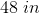.  What is the area?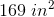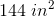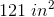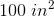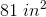Explanation:

The perimeter of a square is given by,  or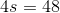. Divide by four to get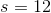.

The area of a square is given by. Substitute the value obtained from the perimeter equation to get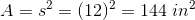.

### Example Question #7 : How To Find The Area Of A Square

The perimeter of a square is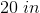.  What is the area?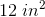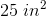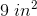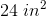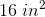Explanation:

The perimeter of a square is given by,  or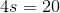. Divide by four to get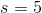.

The area of a square is given by. Substitute the side length obtained from the perimeter equation to get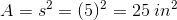.

### Example Question #8 : How To Find The Area Of A Square

What is the area of a square with a side that measuresfeet?square feetsquare feetsquare feetsquare feetsquare feet

Explanation:

The area of a square can be found using the following formula:The side measuresfeet.Therefore, the area of the square issquare feet.

### Example Question #2 : How To Find The Area Of A Square

Becca takes a square napkin and folds it in half once. She then folds it in half again. When she unfolds it, the creases create lines. What best describes the pattern created by the creases?

2 squares

4 squares

2 rectangles

4 rectangles

4 squares

Explanation:

When a napkin is folded in half once, a crease is created vertically down the middle of the napkin. When folded in half once again, another crease is created horizontally across the middle. This creates four squares. Therefore, the best answer is "4 squares."

### Example Question #10 : How To Find The Area Of A Square

A square has a perimeter of 24 inches. What is the area of the square?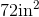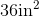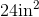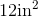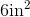Explanation:

Given that all four sides of a square are equal, if the perimeter is 24 inches, then you need to divide by 4 to find the length of each side.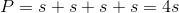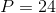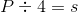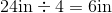The area is found by multiplying two sides together.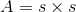Plug in the value ofto solve.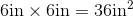Therefore,is the correct answer.

← Previous 1 3 4 5